Refer to our Texas Go Math Grade 4 Answer Key Pdf to score good marks in the exams. Test yourself by practicing the problems from Texas Go Math Grade 4 Module 3 Assessment Answer Key.

Vocabulary

• unit fraction
• equivalent fractions
• factor

Choose the best term from the box.

Question 1.
________________ name the same amount.

Question 2.
A ______________ always has a numerator of 1.

Concepts and Skills

Write two equivalent fractions.

Question 3.
$$\frac{2}{5}$$ = _____________ = ____________

$$\frac{1}{3}$$ = _____________ = ____________

Question 5.
$$\frac{3}{4}$$ = _____________ = ____________

Tell whether the fractions are equivalent. Write = or ≠.

Question 6.
$$\frac{2}{3}$$ ____________ $$\frac{4}{12}$$

Question 7.
$$\frac{5}{6}$$ ____________ $$\frac{10}{12}$$

Question 8.
$$\frac{1}{4}$$ ____________ $$\frac{4}{8}$$

Write the fraction in simplest form.

Question 9.
$$\frac{6}{8}$$

Question 10.
$$\frac{25}{100}$$

$$\frac{8}{10}$$

Write the fraction as a sum of unit fractions.

Question 12.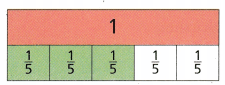$$\frac{3}{5}$$ = ____________

Question 13.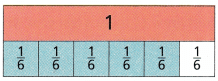$$\frac{5}{6}$$ = ____________

Question 14.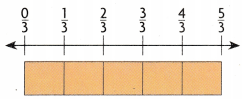$$\frac{5}{3}$$ = ____________

Question 15.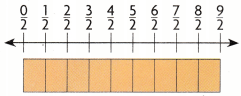$$\frac{9}{2}$$ = ____________

Question 16.
Sam needs $$\frac{5}{6}$$ cup mashed bananas and $$\frac{3}{4}$$ cup mashed strawberries for a recipe. Which shows a pair of fractions that are equivalent to $$\frac{5}{6}$$ and $$\frac{3}{4}$$?
(A) $$\frac{5}{12}$$ and $$\frac{3}{12}$$
(B) $$\frac{10}{12}$$ and $$\frac{2}{12}$$
(C) $$\frac{10}{12}$$ and $$\frac{9}{12}$$
(D) $$\frac{2}{3}$$ and $$\frac{18}{24}$$

Karen will divide her garden into equal parts. She will plant corn in $$\frac{1}{5}$$ of the garden. Which fraction is equivalent to $$\frac{1}{5}$$?
(A) $$\frac{2}{15}$$
(B) $$\frac{3}{12}$$
(C) $$\frac{2}{10}$$
(D) $$\frac{4}{5}$$

Question 18.
Olivia cut a board into 8 equal pieces. She used $$\frac{5}{8}$$ of the pieces.
Which shows $$\frac{5}{8}$$ written as a sum of unit fractions?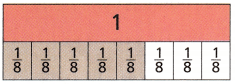(A) $$\frac{1}{8}+\frac{1}{8}+\frac{1}{8}$$
(B) $$\frac{1}{8}+\frac{1}{8}+\frac{1}{8}+\frac{1}{8}+\frac{1}{8}$$
(C) $$\frac{1}{8}+\frac{1}{8}$$
(D) $$\frac{1}{8}+\frac{1}{8}+\frac{1}{8}+\frac{1}{8}$$
Robert grew 1$$\frac{3}{4}$$ inches last year. Which shows 1$$\frac{3}{4}$$ written as a sum of fractions?
(A) $$\frac{1}{4}+\frac{1}{4}+\frac{1}{4}$$
(B) $$\frac{1}{4}+\frac{1}{4}$$
(C) $$\frac{1}{4}+\frac{1}{4}+\frac{1}{4}+\frac{1}{4}+\frac{3}{4}$$
(D) 1 + $$\frac{1}{4}+\frac{1}{4}$$